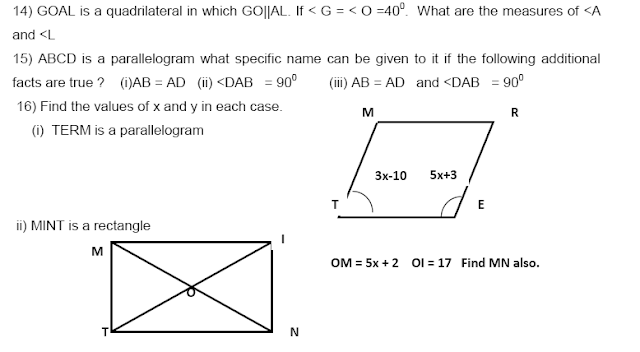# SCC Education

## Understanding Quadrilaterals Practice Paper, Important Questions1. The sum of the angles of a polygon with n – sides is _______________________.
2. The external angle of a regular polygon is 200. How many sides does it have ? What is the measure of each interior angle? What is the total measure of its angles.
3. Is it possible to have a regular polygon with measure of each exterior angle as 580 ? Why? can it be an interior angle of a regular polygon ?
4. Find the measure of each exterior angle of a (i) Regular octagon (ii) Regular Decagon

5. Find the perimeter of a parallelogram with sides 9cm and 5cm.
6. Find the perimeter of a rhombus whose diagonals are 16cm and 12cm
7. The adjacent angles of a parallelogram are in the ratio 5:4 . Find all the angles.
8. If one of the angles of a parallelogram is a right angle, prove that it is a rectangle.
9. If all the angles of a parallelogram are equal. Prove that it is a rectangle.
10. Find the length of the diagonal of a rectangle whose length is 15cm and breadth is 8cm.
11. A square is a convex polygon. Explain why ?
12. The measure of two adjacent angles of a quadrilateral are 1100 and 500 and the other two acute angles are equal. Find the measure of each angle.
13) The five angles of a pentagon are in the ratio 5 : 6 : 7: 8 :10. Find all the angles.formula-sheet

properties-of-parallel-lines

parallelograms properties

volume-and-area-of-cone-and-pyramid

profit-and-loss-questions

compound-interest

volumes-surface-and-area

percentage-and-its-application

ssceducation,scc,education,free notes ,hindi medium notes sharma sir ,9718041826,eco,kuch bhi,ctet,sigma,flower ,on a ,science in hindi ,formula sheet ,notes in pdf ,fee education,maths, science3 ,physics in hindi, general science in hindi,best tutor, 5 star rating center ,grade a ,cbse notes ,test series,mcq,latest science news,in pdf ,doc,ppt, free download ,online solution, free solved notes ,setting,coaching centre ,nda,bird ,class 12,class10 ,class 9,class 11,notes ,